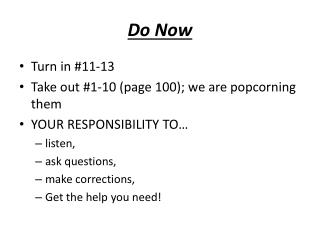Download PresentationDo Now

# Do Now - PowerPoint PPT PresentationDownload Presentation## Do Now

- - - - - - - - - - - - - - - - - - - - - - - - - - - E N D - - - - - - - - - - - - - - - - - - - - - - - - - - -
##### Presentation Transcript

1. Do Now • Turn in #11-13 • Take out #1-10 (page 100); we are popcorning them • YOUR RESPONSIBILITY TO… • listen, • ask questions, • make corrections, • Get the help you need!

2. Look at Car A… m = 1000 kg v = 20 m/s Solve for “p” usingmv= p mv = (1000kg)(20 m/s) mv = 20,000 kg m s • Rank these cars based on momentum before they hit the brakes: • Remember…. m v = p 20,000 kg m s 10,000 kg m s 10,000 kg m s 10,000 kg m s 20,000 kg m s 5,000 kg m s F B = C = D A = E Most NEGATIVE Most POSITIVE

3. Look at Car A… m = 1000 kg Δv = v2 – v1 Δv = 0 – 20 m/s Δv = - 20 m/s mΔv = (1000kg)(-20 m/s) mΔv = -20,000 kg m s In other words… -20,000 N s • Rank these cars based on THE IMPULSE needed to stop them • Remember…. Ft = m Δv - 20,000 N.s -10,000 N.s -10,000 N.s -10,000 N.s -20,000 N.s -5,000 N.s A = E B = C = D F Most NEGATIVE Most POSITIVE

4. Look at Car A… mΔv = Ft -20,000 kg m = Ft s Slight problem…. We don’t know the stopping time! Without ‘t’ we can’t solve for F! • Rank these cars based on THE FORCE needed to stop them • Remember…. Ft = m Δv Not enough info to solve! Most NEGATIVE Most POSITIVE

5. Take out the POGIL that looks like this: • We are checking the LAST PAGE

6. FOLLOW THROUGH in sports Pulling slingshot back all the way Bending knees when jumping Bungee jumping with elastic Running on asphalt Airbags! Moving hand back when catching Head 1st into brick wall Bungee jumping with rope Run on concrete Moving INTO a punch Karate chop Locked knees when jumping

7. Your responsibility to… • Check • Correct • Understand WHY the answer it what it is • Check in with a peer or teacher for help if needed

8. Force x time= Impulse = Momentum Change = massx change in v There are 2 types of algebra problems, combined into one table. Here are your hints when solving: • The 3rd and 4th columns are always equal; that is, the impulse is always equal to the momentum change. • If any two of the first three columns are known, then the remaining column can be computed. • This is true because the impulse=force • time. • Knowing any two of the last three columns allows us to compute the remaining column. • This is true since momentum change = mass • velocity change.

9. - 40 kg m/s Force x time = Impulse = Change in Momentum = mass x change in vel. FIRST ROW - - Do we have enough info to solve for IMPULSE? NO – weonly know time, not force! Do we have enough info to solve for change in momentum? YES! We have mass, and change in velocity! • Change in Momentum = mass x change in vel. • Change in Momentum = 10 kg x (-4 m/s) • Change in Momentum = - 40 kg m/s

10. - 4000 N - 40 Ns - 40 kg m/s Force x time = Impulse = Change in Momentum = mass x change in vel. Do we have enough info to solve for IMPULSE? YES! It equals momentum change! • Impulse = - 40 Ns • Do we have enough info to solve for force? • YES! Force x time = Impulse • Force x (0.01s) = - 40 Ns • Force = - 4000 N

11. - 4000 N - 40 Ns - 40 kg m/s Force x time = Impulse = Change in Momentum = mass x change in vel. - 400 N - 40 kg m/s - 4 m/s 2ND ROW - - Do we have enough info to solve for FORCE? • YES! Force x time = Impulse • Force x (0.1s) = - 40 Ns • Force = - 400 N • Do we have enough info to solve for momentum change? • YES! Impulse = change in momentum! • Do we have enough info to solve for change in velocity? • YES! Change in Momentum = mass x change in vel. • -40 kg m/s = 10 kg x change in vel.

12. - 4000 N - 40 Ns - 40 kg m/s Force x time = Impulse = Change in Momentum = mass x change in vel. - 400 N - 40 kg m/s - 4 m/s - 20,000 -200 - 4 0.01 -200 25 -200 -200 -4 Solutions are above…

13. Exit • Check your chart